Latest Banking jobs   »   Quantitative Aptitude Quiz For IBPS Clerk...

# Quantitative Aptitude Quiz For IBPS Clerk Prelims 2021- 11th November

Directions (1-2): In the following questions, calculate quantity I and quantity II, compare them and answer
(a) If quantity I > quantity II
(b) If quantity I < quantity II
(c) If quantity I ≥ quantity II
(d) if quantity I ≤ quantity II
(e) if quantity I = quantity II or no relation can be established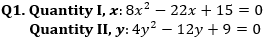Q2. Quantity I, t (in months): A starts a business with an investment of Rs 15000, after t months B joined him with an investment of Rs 20000. At the end of the year the profit share of A and B are Rs 22500 and Rs 17500 respectively.
Quantity II, T (in years): A person invest Rs 18000 at simple interest at rate of 40% per annum for T years. After T years he got interest of Rs 36000.

Directions (3-7): In the following questions, calculate quantity I and quantity II, compare them and answer
(a) If quantity I > quantity II
(b) If quantity I < quantity II
(c) If quantity I ≥ quantity II
(d) if quantity I ≤ quantity II
(e) if quantity I = quantity II or no relation can be established

Q3. Quantity I, time taken by 12 women to complete work alone (in days): 6 men and 9 women working together complete a piece of work in 20 days. The ratio of the efficiencies of a man and a woman is 6 : 5.
Quantity II, time taken by A and B working together to complete work (in days): A alone and B alone can complete the work in 60 days and 84 days respectively.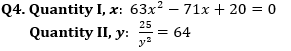Q5. Dharam, Ankit and Harish start a business by investing Rs 7000, Rs 10000 and Rs 12000 respectively. After 4 months Ankit invests Rs 4000 more, after another 4 months Dharam invests Rs 6000 more while Harish withdraws Rs 3000.
Quantity I: Average profit of Dharam, Ankit and Harish after 1 year.
Quantity II: Profit of Harish at the end of the year.

Q6. Quantity I, difference between SI and CI after 2 years: a person invests Rs 12000 each on SI and CI for two years at 20% per annum.
Quantity II, cost price of book: Shopkeeper earns a profit of 12.5% when he sold the book Rs 60 above cost price.

Q7. Quantity I, Distance (in km): A person covers a one third of distance by train with an average speed of 120 kmph, another one third of distance by bus with an average speed of 90 kmph and remaining distance by car with an average speed of 60 kmph. In whole journey he takes 13 hours.
Quantity II: 1200 km

Directions (8-9): The following questions are accompanied by two statements (I) and (II). You have to determine which statements(s) is/are sufficient/necessary to answer the questions.
(a) Statement (I) alone is sufficient to answer the question but statement (II) alone is not sufficient to answer the question.
(b) Statement (II) alone is sufficient to answer the question but statement (I) alone is not sufficient to answer the question.
(c) Both the statements taken together are necessary to answer the question, but neither of the statements alone is sufficient to answer the question.
(d) Either statement (I) or statement (II) by itself is sufficient to answer the question.
(e) Statements (I) and (II) taken together are not sufficient to answer the question.

Q8. P and Q are two workers, if both starts work together and every second day Q works alone, then find time taken to complete the work?

1. Efficiency of Q is 20% more than efficiency of P and working together they can complete the work in 17 days.
2. P and Q started the work and P left after 11 days and remaining work was completed by Q. total time taken in this process to complete the work is 22 days.

Q9. Total no. of male in a village is how much more than total female in year 2018?

1. Total male in 2018 in village is 350 more than total female in village in 2017.
2. Total no. of people in village is 1000 in 2017 and no. of male and female are increased by 25% and 28% respectively in 2018 as compared to 2017.

Directions (10-12): The following questions are accompanied by two statements (I) and (II). You have to determine which statements(s) is/are sufficient/necessary to answer the questions.
(a) Statement (I) alone is sufficient to answer the question but statement (II) alone is not sufficient to answer the question.
(b) Statement (II) alone is sufficient to answer the question but statement (I) alone is not sufficient to answer the question.
(c) Both the statements taken together are necessary to answer the question, but neither of the statements alone is sufficient to answer the question.
(d) Either statement (I) or statement (II) by itself is sufficient to answer the question.
(e) Statements (I) and (II) taken together are not sufficient to answer the question.

Q10. What is the length of the train A?

1. Train A and train B crosses a pole in 21.6 sec and 15 sec respectively. Ratio between speed of train A and train B is 5 : 6.
2. Train A and B crosses each other in 18 sec when they move in opposite direction and when they move in same direction, they cross each other in 3.3 min.

Q11. What is the time taken by Amit to complete the work alone?

1. Hemant alone can complete the work in 40 days and Hemant and Amit working together can complete the same work in 24 days.
2. Deepak alone can complete the work in 80 days. Deepak is 25% less efficient than Amit.

Q12. What is the radius of the cylinder?

1. The total surface area of the cylinder is 3080 cm². The radius of the base of the cylinder and height are in the ratio of 2 : 3.
2. The ratio between the curved surface area and volume of the cylinder is 1 : 7.

Directions (13-15): The following questions are accompanied by two statements (I) and (II). You have to determine which statements(s) is/are sufficient/necessary to answer the questions.
(a) Statement (I) alone is sufficient to answer the question but statement (II) alone is not sufficient to answer the question.
(b) Statement (II) alone is sufficient to answer the question but statement (I) alone is not sufficient to answer the question.
(c) Both the statements taken together are necessary to answer the question, but neither of the statements alone is sufficient to answer the question.
(d) Either statement (I) or statement (II) by itself is sufficient to answer the question.
(e) Statements (I) and (II) taken together are not sufficient to answer the question.

Q13. What is the present age of Shivam.

1. Average of present ages of Dharam, Shivam and Abhishek is 20 years and ratio of their present ages is 5 : 3 : 4 respectively.
2. Abhishek is 5 year older than Shivam. Five years ago, age of Dharam was twice of age of Shivam.

Q14. What is the rate of interest?

1. Shubham invest an amount of Rs 384 at compound interest and after 3 years he got a sum of Rs 750.
2. Amount becomes 3 times of sum if Shubham invests at simple interest for 8 years.

Q15. What is the distance?

1. A man reaches his office late by 12 min when his speed is 50 kmph, if he increases his speed by 22 kmph he will reach his office 10 min early.
2. A man covers half of distance at a speed of 30 kmph and remaining distance at speed of 60 kmph. In this whole journey he takes 1.5 hours.

Solutions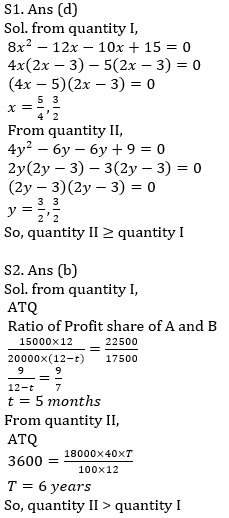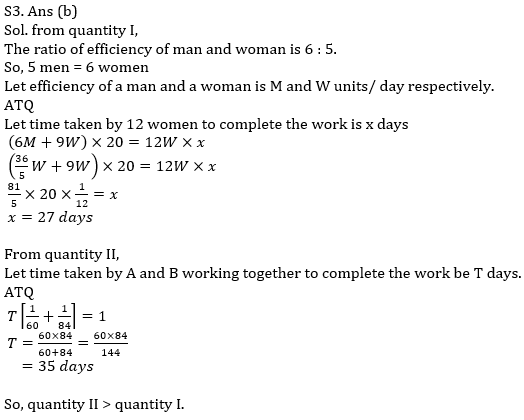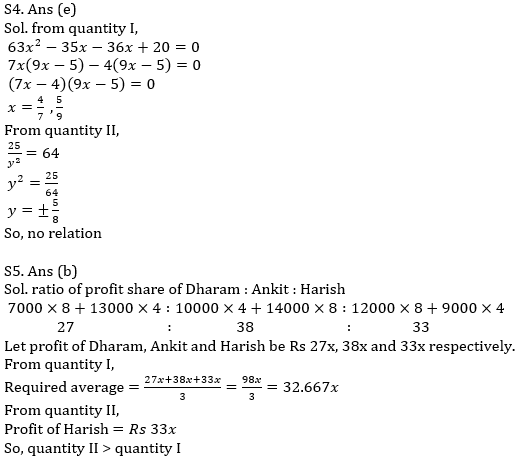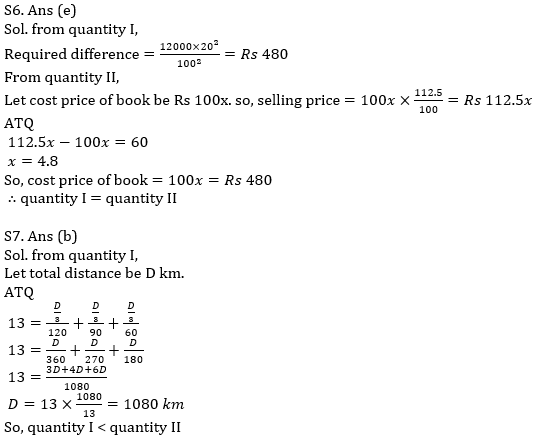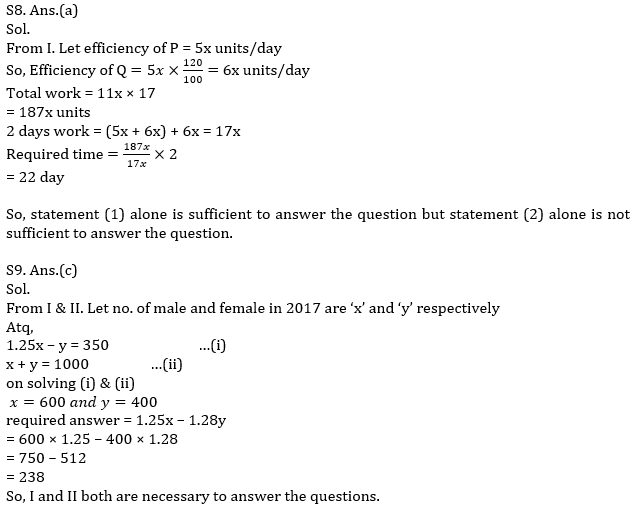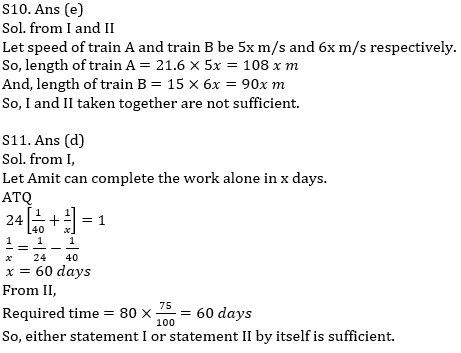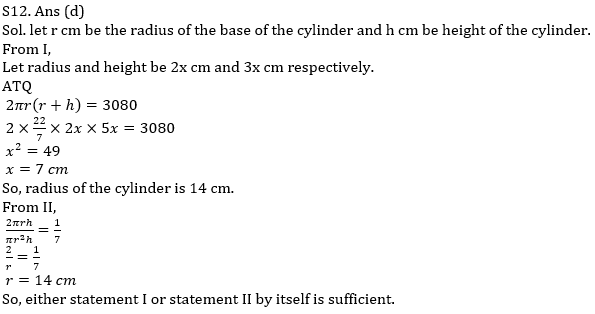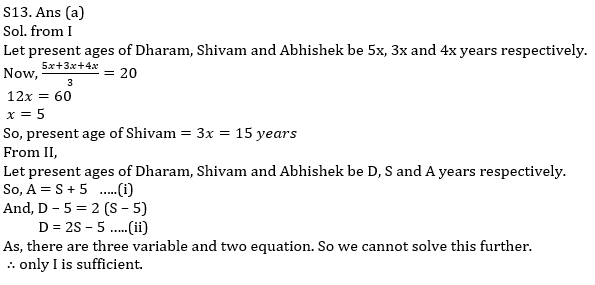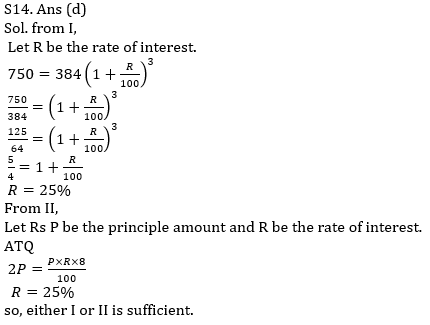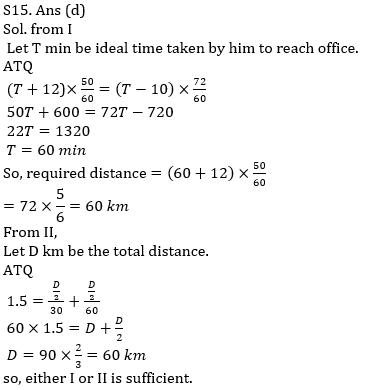×

Thank You, Your details have been submitted we will get back to you.Join India's largest learning destination

What You Will get ?

•Job Alerts
•Daily Quizzes
•Subject-Wise Quizzes
•Current Affairs
•Previous year question papers
•Doubt Solving session

ORJoin India's largest learning destination

What You Will get ?

•Job Alerts
•Daily Quizzes
•Subject-Wise Quizzes
•Current Affairs
•Previous year question papers
•Doubt Solving session

ORJoin India's largest learning destination

What You Will get ?

•Job Alerts
•Daily Quizzes
•Subject-Wise Quizzes
•Current Affairs
•Previous year question papers
•Doubt Solving session

Enter the email address associated with your account, and we'll email you an OTP to verify it's you.Join India's largest learning destination

What You Will get ?

•Job Alerts
•Daily Quizzes
•Subject-Wise Quizzes
•Current Affairs
•Previous year question papers
•Doubt Solving session

Enter OTP

Please enter the OTP sent to
/6

Did not recive OTP?

Resend in 60sJoin India's largest learning destination

What You Will get ?

•Job Alerts
•Daily Quizzes
•Subject-Wise Quizzes
•Current Affairs
•Previous year question papers
•Doubt Solving sessionJoin India's largest learning destination

What You Will get ?

•Job Alerts
•Daily Quizzes
•Subject-Wise Quizzes
•Current Affairs
•Previous year question papers
•Doubt Solving session

Almost there

+91Join India's largest learning destination

What You Will get ?

•Job Alerts
•Daily Quizzes
•Subject-Wise Quizzes
•Current Affairs
•Previous year question papers
•Doubt Solving session

Enter OTP

Please enter the OTP sent to Edit Number

Did not recive OTP?

Resend 60

By skipping this step you will not recieve any free content avalaible on adda247, also you will miss onto notification and job alerts

Are you sure you want to skip this step?

By skipping this step you will not recieve any free content avalaible on adda247, also you will miss onto notification and job alerts

Are you sure you want to skip this step?